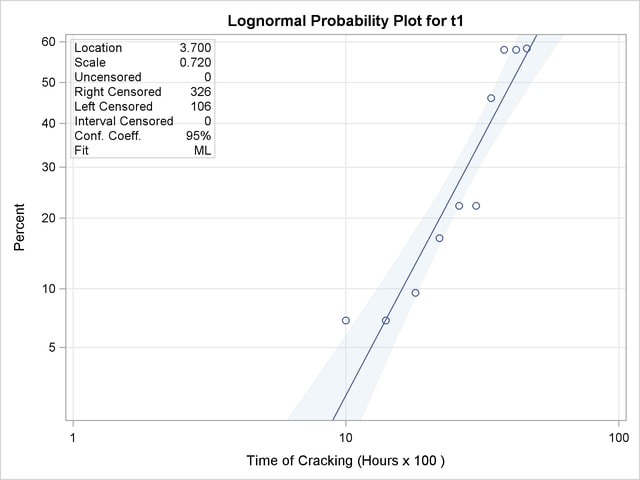The RELIABILITY Procedure
 Lognormal Analysis with Arbitrary Censoring

This example illustrates analyzing data that have more general censoring than in the previous example. The data can be a combination of exact failure times, left censored, right censored, and interval censored data. The intervals can be overlapping, unlike in the previous example, where the interval endpoints had to be the same for all units.

Table 12.2 shows data from Nelson (1982, p. 409), analyzed by Meeker and Escobar (1998, p. 135). Each of 435 turbine wheels was inspected once to determine whether a crack had developed in the wheel or not. The inspection time (in 100s of hours), the number inspected at the time that had cracked, and the number not cracked are shown in the table. The quantity of interest is the time for a crack to develop.

Table 12.2 Turbine Wheel Cracking Data

Inspection Time

Number

Number

(100 hours)

Cracked

Not Cracked

4

0

39

10

4

49

14

2

31

18

7

66

22

5

25

26

9

30

30

9

33

34

6

7

38

22

12

42

21

19

46

21

15

These data consist only of left and right censored lifetimes. If a unit has developed a crack at an inspection time, the unit is left censored at the time; if a unit has not developed a crack, it is right censored at the time. For example, there are 4 left-censored lifetimes and 49 right-censored lifetimes at 1000 hours.

The following statements create a SAS data set named TURBINE that contains the data in the format necessary for analysis by the RELIABILITY procedure:

```   data turbine;
label t1 = 'Time of Cracking (Hours x 100 )';
input t1 t2 f;
datalines;
.   4  0
4   .  39
.  10  4
10  .  49
.  14  2
14  .  31
.  18  7
18  .  66
.  22  5
22  .  25
.  26  9
26  .  30
.  30  9
30  .  33
.  34  6
34  .  7
.  38  22
38  .  12
.  42  21
42  .  19
.  46  21
46  .  15
;
run;
```

The variables T1 and T2 represent the inspection times and determine whether the observation is right or left censored. If T1 is missing (.), then T2 represents a left-censoring time; if T2 is missing, T1 represents a right-censoring time. The variable F is the number of units that were found to be cracked for left-censored observations, or not cracked for right-censored observations at an inspection time.

The following statements use the RELIABILITY procedure to produce the probability plot in Figure 12.15 for the data in the data set TURBINE:

```proc reliability data = turbine;
distribution lognormal;
freq f;
pplot ( t1 t2 ) / maxitem = 5000
ppout;
run;
```

The DISTRIBUTION statement specifies that a lognormal probability plot be created. The FREQ statement identifies the frequency variable F. The option MAXITEM=5000 specifies that the iterative algorithm that computes the points on the probability plot takes a maximum of 5000 iterations. The algorithm does not converge for these data in the default 1000 iterations, so the maximum number of iterations needs to be increased for convergence. The option PPOUT specifies that a table of the cumulative probabilities plotted on the probability plot be printed, along with standard errors and confidence limits.

The tabular output for the maximum likelihood lognormal fit for these data is shown in Figure 12.16. Figure 12.15 shows the resulting lognormal probability plot with the computed cumulative probability estimates and the lognormal fit line.

Figure 12.15 Lognormal Probability Plot for the Turbine Wheel DataFigure 12.16 Partial Listing of the Tabular Output for the Turbine Wheel Data
The RELIABILITY Procedure

Model Information
Input Data Set WORK.TURBINE
Analysis Variable t1 Time of Cracking (Hours x 100 )
Analysis Variable t2
Frequency Variable f
Distribution Lognormal (Base e)
Estimation Method Maximum Likelihood
Confidence Coefficient 95%
Observations Used 21

Cumulative Probability Estimates
Lower Lifetime Upper Lifetime Cumulative
Probability
Pointwise 95% Confidence
Limits
Standard Error
Lower Upper
. 4 0.0000 0.0000 0.0000 0.0000
10 10 0.0698 0.0264 0.1720 0.0337
14 14 0.0698 0.0177 0.2384 0.0473
18 18 0.0959 0.0464 0.1878 0.0345
22 22 0.1667 0.0711 0.3432 0.0680
26 26 0.2222 0.1195 0.3757 0.0657
30 30 0.2222 0.1203 0.3738 0.0650
34 34 0.4615 0.2236 0.7184 0.1383
38 38 0.5809 0.4085 0.7356 0.0865
42 42 0.5809 0.4280 0.7198 0.0766
46 46 0.5836 0.4195 0.7311 0.0822

 Algorithm converged.

Summary of Fit
Observations Used 21
Uncensored Values 0
Right Censored Values 326
Left Censored Values 106
Maximum Loglikelihood -190.7315

Lognormal Parameter Estimates
Parameter Estimate Standard Error Asymptotic Normal
95% Confidence Limits
Lower Upper
Location 3.6999 0.0708 3.5611 3.8387
Scale 0.7199 0.0887 0.5655 0.9165

Other Lognormal Distribution Parameters
Parameter Value
Mean 52.4062
Mode 24.0870
Median 40.4436
Standard Deviation 43.1855Previous Page | Next Page | Top of Page